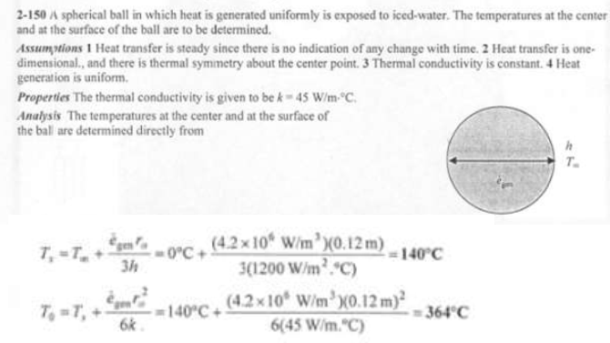heat and mass transfer problems engineering equations heat and mass transfer school homework engineering heat and mass transfer formulas heat and mass transfer solutions to heat and mass transfer problems full solution engineering problem solution heat and mass transfer math problems engineering equations heat and mass transfer school homework engineering solutions to heat and mass transfer formulas heat problems mass problem solutions to transfer problems full solution heat and mass transfer
heat and mass transfer problems engineering equations heat and mass transfer school homework engineering heat and mass transfer formulas heat and mass transfer solutions to heat and mass transfer problems full solution engineering problem solution heat and mass transfer math problems engineering equations heat and mass transfer school homework engineering solutions to heat and mass transfer formulas heat problems mass problem solutions to transfer problems full solution heat and mass transfer
Highalphabet Home Page heat and mass transfer problem solutions Heat and Mass Transfer Page
Heat is generated uniformly at a rate of 4.2x10^6 W/m^3 in a spherical ball (k=45 W/mK) of diameter 24 cm. The ball is exposed to iced-water at 0 C with a heat transfer coefficient of 1200 W/m^2K. Determine the temperatures at the center and the surface of the ball.Heat is generated uniformly at a rate of 4.2x10^6 W/m^3 in a spherical ball (k=45 W/mK) of diameter 24 cm. The ball is exposed to iced-water at 0 C with a heat transfer coefficient of 1200 W/m^2K. Determine the temperatures at the center and the surface of the ball.